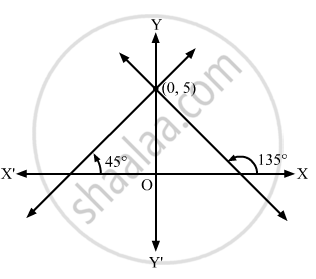Advertisement Remove all ads

# Find the equations of the straight lines which cut off an intercept 5 from the y-axis and are equally inclined to the axes. - Mathematics

Answer in Brief

Find the equations of the straight lines which cut off an intercept 5 from the y-axis and are equally inclined to the axes.

Advertisement Remove all ads

#### Solution

It is given that the lines are equally inclined to the axes.
So, their inclinations with the positive x-axis are ${45}^\circ \text { and } {135}^\circ$.

Let $m_1 \text { and } m_2$ be the slopes of the lines.

$\therefore m_1 = \tan {45}^\circ = 1 \text { and } m_2 = \tan {135}^\circ = - \tan {45}^\circ = - 1$

Thus, the equations of the lines passing through (0, 5) with slopes $1 \text { and }- 1$ are

$y - 5 = 1\left( x - 0 \right) \text { and } y - 5 = - 1\left( x - 0 \right)$

$\Rightarrow y - x - 5 =\text { and } y + x - 5 = 0$

$\Rightarrow y = x + 5 \text { and }x + y = 5$Is there an error in this question or solution?
Advertisement Remove all ads

#### APPEARS IN

RD Sharma Class 11 Mathematics Textbook
Chapter 23 The straight lines
Exercise 23.4 | Q 8 | Page 29
Advertisement Remove all ads

#### Video TutorialsVIEW ALL 

Advertisement Remove all ads
Share
Notifications

View all notifications

Forgot password?